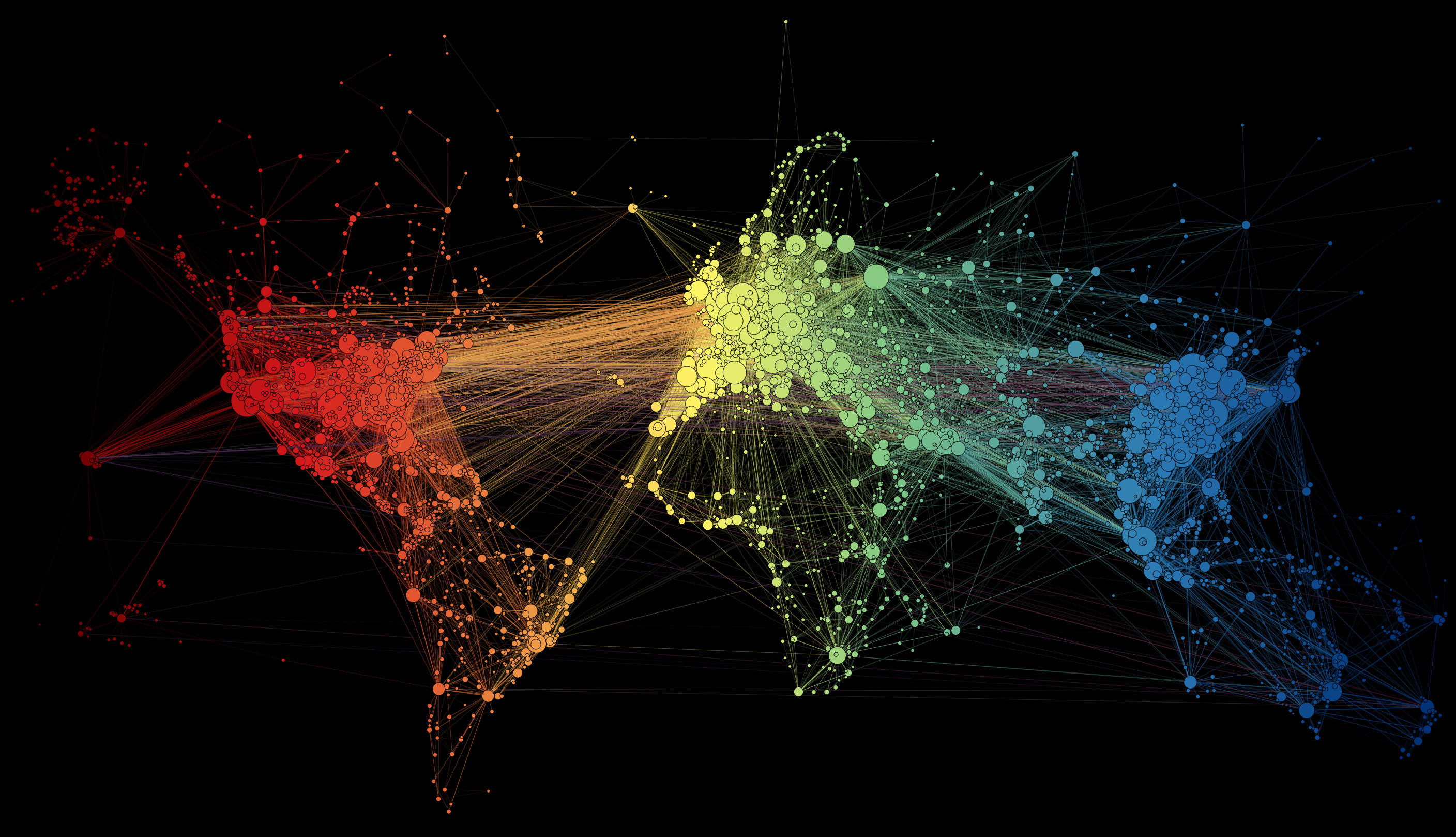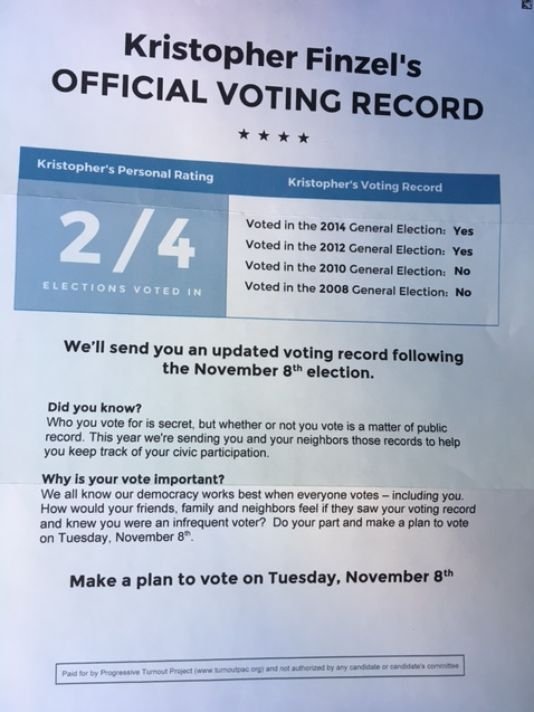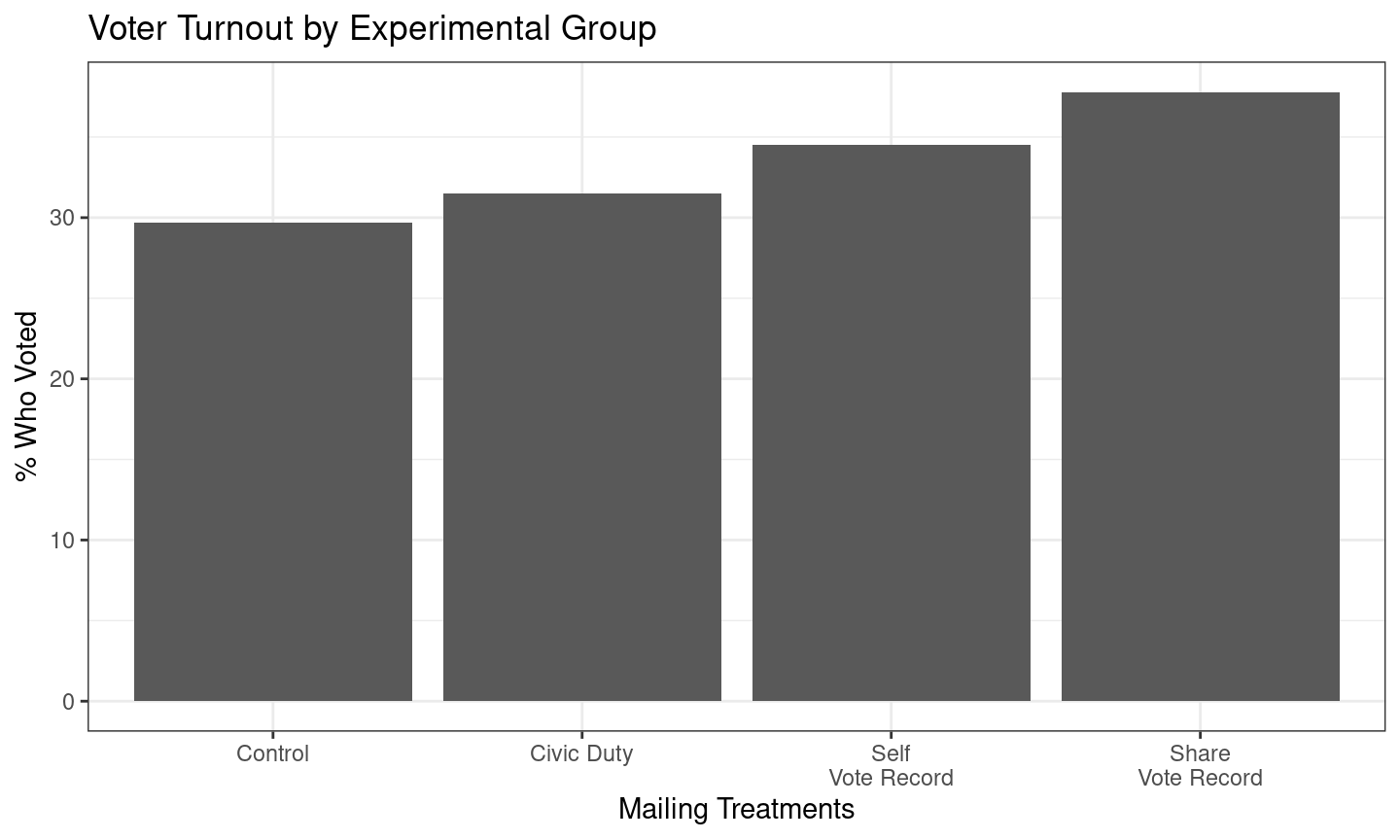March 9, 2021

## Objectives

### 1. What is Causality

• Counterfactuals
• Potential Outcomes

### 2. Types of causal claims

• deterministic vs probabilistic
• varieties of deterministic causal claims

## A question for today:

From the midterm, and still a relevant policy consideration:

Do public health polices that require individuals to wear face-masks in public indoor spaces reduce COVID transmission and mortality?

• This is a causal question.

## What is causality?

All causal claims are counterfactual claims:

That is: they imply two descriptive claims of a specific type

1. One descriptive claim about how the world is factually;
• what is the factual exposure to the alleged “cause”
• what is the factual outcome (the thing allegedly affected)
2. One descriptive claim about how the world would be counterfactually
• what would the outcome be if exposure to the alleged cause were different

## Counterfactuals and Causality:

counterfactuals: are the way world would be if events had transpired differently (other than what actually took place).

• imagine an “alternate universe”

constrasts to what is factual: the way the world is, given the events that have taken place.

## Counterfactuals and Potential Outcomes

Counterfactuals imply potential outcomes:

If $$X$$ is a variable that corresponds to a possible cause and $$Y$$ is a variable for what is possibly affected, and $$i$$ indicates a specific case…

then potential outcomes are the values of $$Y_i$$ a specific case would take for the different possible values of $$X$$ (both factual and counterfactual)

• potential outcomes because they could happen but only one becomes factual, the others remain counterfactual.

## Counterfactuals and Potential Outcomes

$$Y_i$$ = COVID Deaths in a Canadian Province

$$X$$ = Mandatory Face-mask Policy (yes or no)

Only one policy will be implemented; COVID deaths in the alternate world remain counterfactual (what would have happened absent face-mask policy).

## Counterfactuals and Causality:

And we can say that $$X$$ causes $$Y$$ for case $$i$$ if $$Y_i(X = 1) \neq Y_i(X = 0)$$:

• $$X$$ causes $$Y$$: if case $$i$$ would have behaved ($$Y$$) differently (than it did factually) in the (counterfactual) alternate universe where everything was the same except for $$X$$.

• Same as saying: $$X$$ causes $$Y$$ if the potential outcomes of $$Y$$ are different for different values of $$X$$

## Counterfactuals and Causality:

And we can say that $$\mathrm{Face \ Mask \ Policy}$$ reduced $$\mathrm{COVID \ Deaths}$$ for case $$BC$$ if

$$\mathrm{COVID \ Deaths}_{BC}(\mathrm{Mask = Yes}) <$$ $$\mathrm{COVID \ Deaths}_{BC}(\mathrm{Mask = No})$$:

## Counterfactuals and Causality:

All causal claims can be re-stated as counterfactual claims

• They contain a conditional clause, starting with “If” (always in the subjunctive mood)
• A “then” clause, stating what would happen if the conditional/“If” clause were true (always in the conditional mood)
• May be in past, present, or future tense.

## Counterfactuals and Causality:

### Example: Past

“BC’s mask mandate reduced COVID fatalities.”

$\underbrace{If \ \ BC \ \ had \ \ not \ \ imposed \ \ a \ \ mask \ \ mandate}_{\text{If-clause in Subjunctive Mood}}, \\ \underbrace{there \ \ would \ \ have \ \ been \ \ more \ \ COVID \ \ deaths.}_{\text{Then-clause in Conditional Mood}}$

## Counterfactuals and Causality:

### Example: Present

“The presence of greek organizations on campus increases rates of sexual assault.”

$\underbrace{If \ \ there \ \ were \ \ no \ \ greek \ \ organizations \ \ on \ \ campus}_{\text{If-clause in Subjunctive Mood}}, \\ \underbrace{there \ \ would \ \ be \ \ fewer \ \ sexual \ \ assaults.}_{\text{Then-clause in Conditional Mood}}$

## Counterfactuals and Causality:

Note: Counterfactual claims get increasingly complicated, the more complicated your causal claim is

• On assignments, exam do not come up with overly complex causal claims.

## Two ways of asking causal questions

### What are the causes of effects?

Want to explain something specific that has happened/we observe (the effect). Seek to attribute a cause for something we observe.

• “Why has Quebec had the highest rate of COVID mortality among all provinces in Canada?”

## Two ways of asking causal questions

### What are the effects of causes?

We want to know what happens if we do some action or some action (the cause) happens. (Could be a specific action or not) This is about the contribution of some cause to an effect.

• What is the effect of the Canada Emergency Response Benefit (CERB) on consumer spending?

### In groups

1. Come up with two causal claims; one corresponding each of the preceding two questions

2. Restate each causal claim as a counterfactual claim.

## Two ways of asking causal questions

Usually… different kinds of causal questions are answered with different kinds of causal claims

1. causes of effects $$\to$$ deterministic causal claims
2. effects of causes $$\to$$ probabilistic causal claims

## Deterministic Causal Claims

deterministic causal claims

• claims about what happenens with certainty under specific causal conditions
• whenever some cause (or set of causes) is present, the effect always happens
• if the cause is present the effect is determined (unavoidable)
• usually make these claims when we are interested in causes of effects

## Deterministic Causal Claims

There are several varieties and combinations

• necessary conditions
• sufficient conditions
• conjunctural/multiple causation

## Necessary Conditions

### necessary conditions

A causal claim that there is some cause $$C$$ without which the effect cannot occur

• A cause $$C$$ must happen in order for effect $$E$$ to happen.
• Does not mean if the cause $$C$$ is present, effect $$E$$ must happen, only without $$C$$, no $$E$$.

## Necessary Conditions: Example

A claim: “Without global air travel, a new infectious disease will not become a global pandemic.”

Also can be stated: “Global air travel is a necessary condition for a new disease to become a pandemic.”

## Map of Air Travel in 2015POLL

## Necessary Conditions: Example

If this claim is true: “Global air travel is a necessary condition for a new disease to become a pandemic.”…

Does the graph on preceding page imply that a new infectious disease will become a pandemic?

• No. Presence of necessary condition $$\not\to$$ effect must happen. Instead, absence of necessary condition $$\to$$ effect does not happen

## Sufficient Conditions

(In contrast to necessary conditions)

### sufficient conditions

• cause $$C$$ always produces an effect $$E$$ when it is present
• do not depend on other factors being present; cause $$C$$ can produce $$E$$ by itself
• Sufficient conditions imply: every time $$C$$ is present, then $$E$$ will happen

## Sufficient Conditions: Example

“A military coup that overthrows a democratically elected government is a sufficient condition for large public protests.”

• This might be the case every time
• Does not appear to depend on other factors

## Things are usually more complicated

### BC vs. Washington State

Since the pandemic has begun, BC has had a 27.4 COVID deaths per 100000 people. Washington State, directly across the US border to the south, has had 67.5 COVID deaths per 100000 people.

In your group, propose an answer: What caused BC to have a lower COVID death rate than Washington State?

## Complex Causality

Causality may be deterministic… there are exact conditions for when effect always/never happens.

But in reality, it is almost always complex

• multiple factors might be necessary (conjunctural)
• different causes produce same effect (multiple)
• different groups of factors might, together be sufficient (multiple and conjunctural)
• (INUS/SUIN conditions: see here)

## Voting Experiment

### Why do people vote?## Voting ExperimentPOLL

## Complex Causality

Does it make sense to say that “being shamed” is a necessary condition for voting?

• No. Clearly some people voted in absence of shaming

Does it make sense to say that “being shamed” is a sufficient condition for voting?

• No. Some people were shamed but did not vote.
• It is simpler to state this probabilistically: being shamed increases likelihood of voting.

## Probabilistic Causal Claims

### probabilistic causal claims

are claims that the presence/absence of a cause $$C$$ makes an effect $$E$$ more or less likely to occur. Or cause $$C$$ increases/decreases effect $$E$$ on average

• In contrast to deterministic causal claims this implies
• effect $$E$$ can happen when $$C$$ is absent
• effect $$E$$ may not happen when $$C$$ is present
• NOT a claim that politics has some inherent randomness (e.g. quantum mechanics)
• Usually make these claims when interested in effects of causes